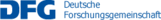## C09 – Adaptive methods for high-dimensional eigenvalue problems and their computational complexity

The subject of this project are numerical methods for high-dimensional eigenvalue problems of partial differential operators and their computational complexity. For obtaining approximations that exploit structural features of the eigenfunctions of interest, our focus lies on the combination of adaptive discretisations of the considered operators with low-rank tensor decompositions of coefficient sequences. We build on recent advances for linear operator equations, where methods that build such highly nonlinear approximations at near-optimal computational complexity have been constructed, and we aim to achieve comparable results for eigenvalue problems.

An application of particular interest are Schrödinger equations in quantum physics. Here one additionally needs to account for symmetry or antisymmetry of wave functions, which in the context of low-rank tensor approximations can be done in a second quantisation representation in terms of occupation numbers with respect to single-particle orbitals. Established solvers for high-dimensional occupation number tensors which alternate between tensor representation components, such as density matrix renormalisation group algorithms, are known to fail in certain situations. The problem of constructing methods with convergence and complexity guarantees becomes especially interesting when the underlying orbitals are optimised to yield the most efficient low-rank decomposition, which in the quantum chemistry context amounts to a generalisation of multi-configuration self-consistent field approximations.

In the latter setting, orbitals need to be represented with respect to a set of reference basis functions. The adaptive identification of such sparse representations requires new a posteriori error estimation techniques, which we study for low-rank approximations of general eigenvalue problems on product domains. A closely related issue is preconditioning with low-rank representations and the appropriate treatment of norms on the relevant function spaces, where certain incompatibilities between energy norms and tensor structures need to be addressed.

Name Institute Location Phone
Bachmayr, MarkusU Mainzbachmayr@uni-mainz.de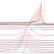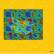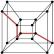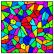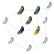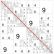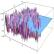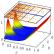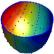## Contact

Coordinator: Prof. Dr. Stefan Müller